Tuesday, 03 September 2013 02:08

### Background

Excel is widely used to organize data manipulations like arithmetic operations. Excel provides many built-in functions which automate a number of types of calculation. Functions are pre-programmed formulate for example, the square-root function, trigonometric functions, logarithms etc. Excel has more than 300 functions covering a range of statistical, mathematical, financial and logical operations. There is no doubt that using a function offers a shortcut method.

### Calculate Formulas in XLS Document

Microsoft Excel is a powerful tool which has many uses, the most basic feature of which is performing functions. The aim of this article is to help you perform simple arithmetic operations on values in programming by using excel functions. Spire.Xls for .NET can help you easily create a new excel document or load an existing excel document into program, and calculate data of designated cell by function. Applied in Console platform, WinForm and Asp.net, It provide different types of mathematical functions, statistical functions , logic functions ,and string functions to calculate data with C# codes.

The following is the method example of using Console application to show how Spire.XLS for .NET realizes the calculation formula:

Step 1: Build a console application, and add spire.XLS.dll, Spire.Common.dll assembly.

Step 2: Instantiate an object of Spire.Xls.WorkBook, and add a “WorkSheet” in WorkBook object.

[C#]
```Workbook workbook = new Workbook();
Worksheet sheet = workbook. Worksheets;
```

Step 3: Set the value and format in Cell A1 and Cell A3.veiwing the C# Code.

[C#]
```//set Column A, B, C width
sheet.SetColumnWidth(1, 32);
sheet.SetColumnWidth(2, 16);
sheet.SetColumnWidth(3, 16);

// Set value of Cell A1
sheet.Range[currentRow++, 1].Value = "Examples of formulas :";
// Set value of Cell A2.
sheet.Range[++currentRow, 1].Value = "Test data:";

// Set text format Of Cell A1
CellRange range = sheet.Range["A1"];
range.Style.Font.IsBold = true;
range.Style.FillPattern = ExcelPatternType.Solid;
range.Style.KnownColor = ExcelColors.LightGreen1;
range.Style.Borders[BordersLineType.EdgeBottom].LineStyle = LineStyleType.Medium;
```

Step 4: Set some cells value and then to sum up some cells data and the results will be displayed in one of the cells.

[C#]
```sheet.Range[currentRow, 2].NumberValue = 7.3;
sheet.Range[currentRow, 3].NumberValue = 5;
sheet.Range[currentRow, 4].NumberValue = 8.2;
sheet.Range[currentRow, 5].NumberValue = 4;
sheet.Range[currentRow, 6].NumberValue = 3;
sheet.Range[currentRow, 7].NumberValue = 11.3;
//Create arithmetic expression string about cells

currentFormula = "=Sheet1!\$B\$3 + Sheet1!\$C\$3+Sheet1!\$D\$3+Sheet1!\$E\$3+Sheet1!\$F\$3+Sheet1!\$G\$3";
formulaResult = workbook.CaculateFormulaValue(currentFormula);
value = formulaResult.ToString();
sheet.Range[currentRow, 2].Value = value;
```

Step 5: Respectively set value and text format of Cell A4, B4.

[C#]
```sheet.Range[++currentRow, 1].Value = "Formulas"; ;
sheet.Range[currentRow, 2].Value = "Results";
range = sheet.Range[currentRow, 1, currentRow, 2];
range.Style.Font.IsBold = true;
range.Style.KnownColor = ExcelColors.LightGreen1;
range.Style.FillPattern = ExcelPatternType.Solid;
range.Style.Borders[BordersLineType.EdgeBottom].LineStyle = LineStyleType.Medium;
```

Step 6: Realize calculation simple expression.

[C#]
```// Create arithmetic tables enclosed type string
currentFormula = "=33*3/4-2+10";
sheet.Range[++currentRow, 1].Text = currentFormula;
// Caculate arithmetic expression
formulaResult = workbook.CaculateFormulaValue(currentFormula);
value = formulaResult.ToString();
sheet.Range[currentRow, 2].Value = value;
```

Step 7: Realize some mathematic functions.

[C#]
```//absolute value function .
currentFormula = "=ABS(-1.21)";
sheet.Range[currentRow, 1].Text = currentFormula;
sheet.Range[currentRow++, 2].Formula = currentFormula;
```

Step 8: Realize some logic function.

[C#]
```//NOT function
//Create NOT function string
currentFormula = "=NOT(true)";
sheet.Range[currentRow, 1].Text = currentFormula;
//Caculate NOT function
formulaResult = workbook.CaculateFormulaValue(currentFormula);
value = formulaResult.ToString();
sheet.Range[currentRow, 2].Value = value;
sheet.Range[currentRow, 2].HorizontalAlignment = HorizontalAlignType.Right;
```

Step 9: Realize some string handling functions.

[C#]
```//Get the substring
// Build substring function
currentFormula = "=MID(\"world\",4,2)";
sheet.Range[++currentRow, 1].Text = currentFormula;
//Caculate substring function
formulaResult = workbook.CaculateFormulaValue(currentFormula);
value = formulaResult.ToString();
sheet.Range[currentRow, 2].Value = value;
sheet.Range[currentRow, 2].HorizontalAlignment = HorizontalAlignType.Right;
```

Step 10: Realize a random function.

[C#]
```// Random function
// Create random function string.
currentFormula = "=RAND()";
sheet.Range[++currentRow, 1].Text = currentFormula;
//Caculate random function
formulaResult = workbook.CaculateFormulaValue(currentFormula);
value = formulaResult.ToString();
sheet.Range[currentRow, 2].Value = value;
```

Step 11: Save workbook object as file.

[C#]
```workbook.SaveToFile("formulaTest.xls",ExcelVersion.Version97to2003);
```

Viewing the full c# code

[C#]
```using System;
using System.Collections.Generic;
using System.Text;
using Spire.Xls;

namespace XlsCalculateFormula
{
class Program
{
static void Main(string[] args)
{
//Instanitate an object of Spire.Xls.Workbook
Workbook workbook = new Workbook();
// Add a Spire.Xls.Worksheet to Spire.Xls.Workbook
Worksheet sheet = workbook.Worksheets;

int currentRow = 1;
string currentFormula = string.Empty;
object formulaResult = null;
string value = string.Empty;

// Set width respectively of Column A ,Column B,Column C
sheet.SetColumnWidth(1, 32);
sheet.SetColumnWidth(2, 16);
sheet.SetColumnWidth(3, 16);

//Set the value of Cell A1
sheet.Range[currentRow++, 1].Value = "Examples of formulas :";
// Set the value of Cell A2
sheet.Range[++currentRow, 1].Value = "Test data:";
// Set the style of Cell A1
CellRange range = sheet.Range["A1"];
range.Style.Font.IsBold = true;
range.Style.FillPattern = ExcelPatternType.Solid;
range.Style.KnownColor = ExcelColors.LightGreen1;
range.Style.Borders[BordersLineType.EdgeBottom].LineStyle = LineStyleType.Medium;

// Additive operation of mutiple cells
sheet.Range[currentRow, 2].NumberValue = 7.3;
sheet.Range[currentRow, 3].NumberValue = 5; ;
sheet.Range[currentRow, 4].NumberValue = 8.2;
sheet.Range[currentRow, 5].NumberValue = 4;
sheet.Range[currentRow, 6].NumberValue = 3;
sheet.Range[currentRow, 7].NumberValue = 11.3;
// Create arithmetic expression string about cells
currentFormula = "=Sheet1!\$B\$3 + Sheet1!\$C\$3+Sheet1!\$D\$3+Sheet1!\$E\$3+Sheet1!\$F\$3+Sheet1!\$G\$3";
formulaResult = workbook.CaculateFormulaValue(currentFormula);
value = formulaResult.ToString();
sheet.Range[currentRow, 2].Value = value;

// Set the value and format of two head cell
sheet.Range[++currentRow, 1].Value = "Formulas"; ;
sheet.Range[currentRow, 2].Value = "Results";
sheet.Range[currentRow, 2].HorizontalAlignment = HorizontalAlignType.Right;
range = sheet.Range[currentRow, 1, currentRow, 2];
range.Style.Font.IsBold = true;
range.Style.KnownColor = ExcelColors.LightGreen1;
range.Style.FillPattern = ExcelPatternType.Solid;
range.Style.Borders[BordersLineType.EdgeBottom].LineStyle = LineStyleType.Medium;

// Expression caculation

// Create arithmetic tables enclosed type string
currentFormula = "=33*3/4-2+10";
sheet.Range[++currentRow, 1].Text = currentFormula;
// Caculate arithmetic expression
formulaResult = workbook.CaculateFormulaValue(currentFormula);
value = formulaResult.ToString();
sheet.Range[currentRow, 2].Value = value;

/// The mathematics function ///

//Absolute value function

// Create abosolute value function string
currentFormula = "=ABS(-1.21)";
sheet.Range[++currentRow, 1].Text = currentFormula;
// Caculate abosulte value function
formulaResult = workbook.CaculateFormulaValue(currentFormula);
value = formulaResult.ToString();
sheet.Range[currentRow, 2].Value = value;

///  Statistical function///

// Sum function
// Create sum function string
currentFormula = "=SUM(18,29)";
sheet.Range[++currentRow, 1].Text = currentFormula;
// Caculate sum function
formulaResult = workbook.CaculateFormulaValue(currentFormula);
value = formulaResult.ToString();
sheet.Range[currentRow, 2].Value = value;

///logic function///

//NOT function
// Create NOT function string
currentFormula = "=NOT(true)";
sheet.Range[currentRow, 1].Text = currentFormula;
//Caculate NOT function
formulaResult = workbook.CaculateFormulaValue(currentFormula);
value = formulaResult.ToString();
sheet.Range[currentRow, 2].Value = value;
sheet.Range[currentRow, 2].HorizontalAlignment = HorizontalAlignType.Right;

///String Manipulation function///
//Get the substring
// Build substring function
currentFormula = "=MID(\"world\",4,2)";
sheet.Range[++currentRow, 1].Text = currentFormula;
//Caculate substring function
formulaResult = workbook.CaculateFormulaValue(currentFormula);
value = formulaResult.ToString();
sheet.Range[currentRow, 2].Value = value;
sheet.Range[currentRow, 2].HorizontalAlignment = HorizontalAlignType.Right;

// Random function
// Create random function string.
currentFormula = "=RAND()";
sheet.Range[++currentRow, 1].Text = currentFormula;
//Caculate random function
formulaResult = workbook.CaculateFormulaValue(currentFormula);
value = formulaResult.ToString();
sheet.Range[currentRow, 2].Value = value;

// Save Spire.Xls.Workbook as exel file
workbook.SaveToFile("formulaTest2.xls",ExcelVersion.Version97to2003);
System.Diagnostics.Process.Start("formulaTest2.xls");
}
}
}
```

### Screenshot: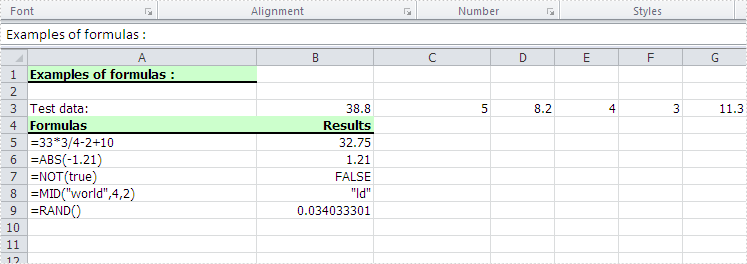Thursday, 22 August 2013 08:41

### Background

This technical article mainly discuss the method that how Spire.Doc fulfill its printing function. Word document developed by Microsoft is now widely used for office users. It is a common need when people need to print word document through a printer. While for programmers, it would not be a simple task to load word document into program and pint it out. Especially when it comes to web printing, it is difficult to realize background printing without interaction.

Aimed at these two points, we developed Spire.Doc for .NET, by which you can easily load word document into your .NET applications, and print it entirely out. In fact, it can be applied in console platform, WinForm, and Asp.net application.

### Approach

The following method is based on the example of console platform. Firstly, you can click here to download Spire.Doc for .NET. Below is the screenshot of the original word document: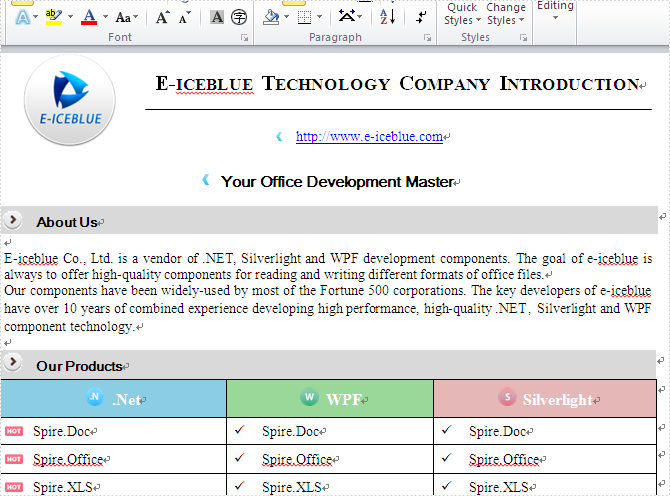Step1: Create a new project in Visual Studio. Then set its target framework to be .NET framework 2.0. Add reference assembly "System.windows.forms.dll, System.Drawing.dll, Spire.Doc.dll"

Step2: Instantiated an object of Spire.Doc.Document and call its method of LoadFromFile in your project to load Word document.

[C#]
```Document doc = new Document();
```

Step3: Instantiated an object of System.Windows.Forms.PrintDialog and set some of its relative properties. Here, one key point has to be noticed that you should associate System.Windows.Forms.PrintDialog object with Spire.Doc.Document while in this process.

[C#]
```PrintDialog dialog = new PrintDialog();
dialog.AllowPrintToFile = true;
dialog.AllowCurrentPage = true;
dialog.AllowSomePages = true;
dialog.UseEXDialog = true;
doc.PrintDialog = dialog;
```

Step4: Start background printing. You should get System.Drawing.Printing.PrintDocument object by using PrintDocument properties of Spire.Doc.Document. Now you can print entire pages with default printer without interaction. (This printing mode is also suitable for Web printing)

[C#]
```PrintDocument printDoc = doc.PrintDocument;
printDoc.Print();
```

Step5: Implement interaction printing.Call the ShowDialog method of System.Windows.Forms.PrintDialog to print word document

[C#]
```if (dialog.ShowDialog() == DialogResult.OK)
{
printDoc.Print();
}
```

The following screenshot reveals the result after printing word document to XPS format: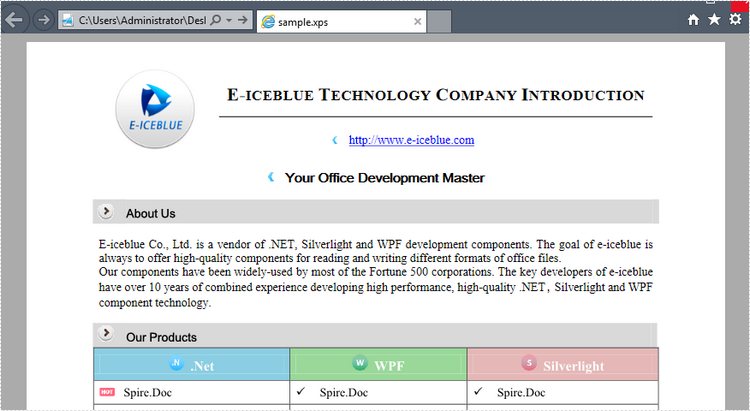This is the complete c# code throughout this process:

[C#]
```using System;
using System.Collections.Generic;
using System.Text;
using Spire.Doc;
using System.Windows.Forms;
using System.Drawing.Printing;

namespace Doc_Print
{
class Program
{
static void Main(string[] args)
{
// Instantiated an object of Spire.Doc.Document
Document doc = new Document();
// Instantiated System.Windows.Forms.PrintDialog object .
PrintDialog dialog = new PrintDialog();
dialog.AllowPrintToFile = true;
dialog.AllowCurrentPage = true;
dialog.AllowSomePages = true;
dialog.UseEXDialog = true;
// associate System.Windows.Forms.PrintDialog object with Spire.Doc.Document
doc.PrintDialog = dialog;
PrintDocument printDoc = doc.PrintDocument;
//Background printing
printDoc.Print();
//Interaction printing
if (dialog.ShowDialog() == DialogResult.OK)
{
printDoc.Print();
}

}
}
}
```
Thursday, 08 August 2013 07:38

### How to Create PDF Dynamically and Send it to Client Browser Using ASP.NET?

Portable Document Format (PDF)is a fixed-layout document as an independent specification by Adobe. It encapsulates a complete description including the text fonts, graphics, and other information needed to display it.

### Create PDF dynamically and send it to client browser

To generate a PDF file dynamically and then send it to client browser, you can use Spire.PDF for .NET to finish this task. In addition, Spire.PDF supports loading an existing PDF file and send it to client browser as well. In this technical article, we will combine both two functions to made a fully description about how Spire.PDF make it work. Below are the two tasks:

• Task1 Create PDF dynamically and send it to client browser.
• Task2 Load an Existing PDF file and send it to client browser(This is an additional function of Spire.PDF for .NET)

Firstly create an Asp.net application and add Spire.PDF.dll assembly. You can add two buttons on an Aspx page in VS. Appoint one of them for Task 1, and the other for Task 2.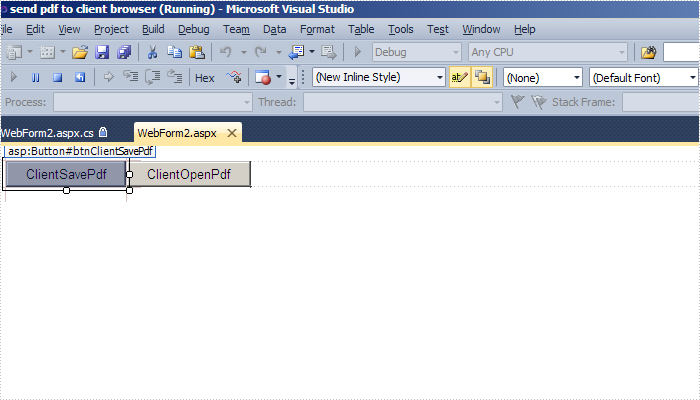In terms of Task 1, firstly you need initiate an object of Spire.PdfDocument

[C#]
```PdfDocument doc = new PdfDocument();
```

And add a new page in this new PDF document

[C#]
```PdfPageBase page = newDoc.Pages.Add();
```

Pay attention that related auxiliary object are need while drawing string on this pdf page.

[C#]
```string message = "Hello world!";
PdfFont font = new PdfFont(PdfFontFamily.Helvetica, 13f);
PdfBrush brush = PdfBrushes.Red;
PointF location = new PointF(20, 20);
```

Then you can draw a string in pdf page, something like this:

[C#]
```page.Canvas.DrawString(message, font, brush, location);
```

Finally you can open this newly generated PDF document in Client browser:

[C#]
```newDoc.SaveToHttpResponse("sample.pdf",HttpContext.Current.Response, HttpReadType.Open);
```

When it comes to Task 2, 3 lines of code can work it out directly.

Initiated an object of Spire.PdfDocument

[C#]
```pdfDocument doc = new PdfDocument();
```

[C#]
```doc.LoadFromFile(this.Server.MapPath("/sample.pdf"));
```

Load a pdf document ,after that ,send it to client browser as an attachment.

[C#]
```doc.SaveToHttpResponse("sample.pdf", this.Response, HttpReadType.Save);
```

To summarize above, the following is the complete code snippet needed in this two tasks:

[C#]
```C#
using System;
using System.Collections.Generic;
using System.Drawing;
using System.Web;
using System.Web.UI;
using System.Web.UI.WebControls;
using Spire.Pdf;
using Spire.Pdf.Graphics;

namespace SendPdfToWebBrowser
{
public partial class WebForm_SendPdf : System.Web.UI.Page
{
protected void Page_Load(object sender, EventArgs e)
{

}
// load a pdf document ,after that ,send it to client browser as an attachment
protected void btnClientSavePdf_Click(object sender,EventArgs e)
{

// initiated an object of Spire.PdfDocument
PdfDocument doc = new PdfDocument();

// send the pdf document to client browser as an attachment
}

// Create an pdf document ,then open it in the client browser
protected void btnClientOpenPdf_Click(object sender, EventArgs e)
{
// Initiate an object of Spire.PdfDocument
PdfDocument newDoc = new PdfDocument();
// Add a new page in this newly created pdf file
string message = "Hello world!” ;

PdfFont font = new PdfFont(PdfFontFamily.Helvetica,13f);
PdfBrush brush = PdfBrushes.Red;
PointF location = new PointF(20, 20);
// Draw a string with designated brush, a font, position in pdf page
page.Canvas.DrawString(message, font, brush, location);
//To open this pdf document in client browser.
}
}
}
```

In the end, you can run it, and get things like this: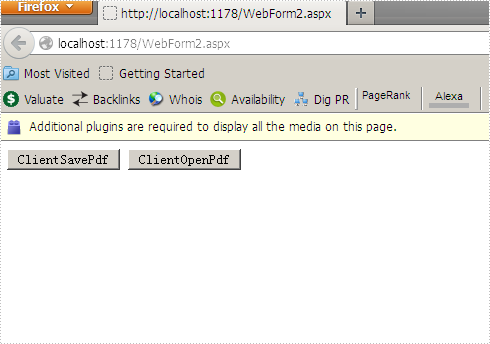Screenshot of creating PDF dynamically and sending it to client browser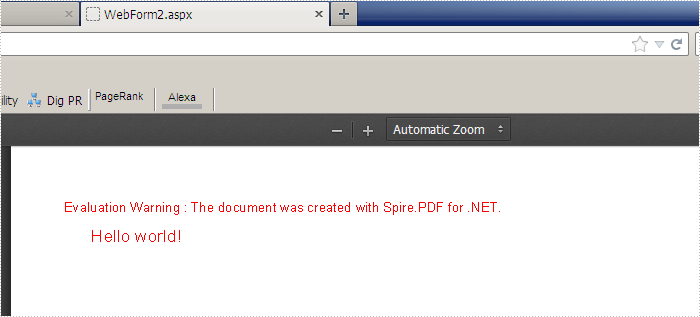Screenshot of Loading an Existing PDF file and sending it to client browser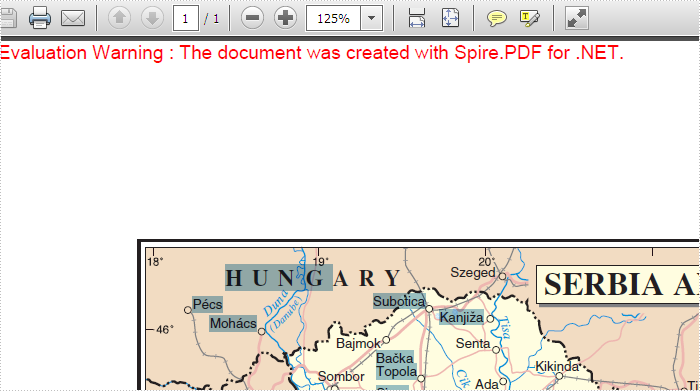Monday, 05 March 2012 07:29

### Export PDF Document to images

The sample demonstrates how to export PDF pages as images by PdfDocumentViewer Component.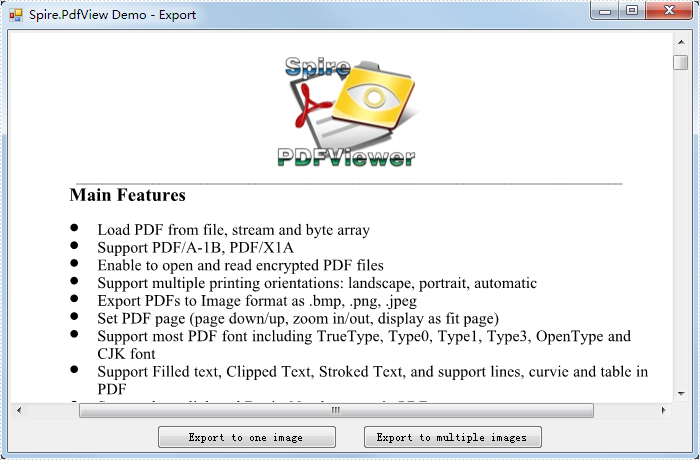Tuesday, 30 August 2011 09:21

### XLS Report Silverlight

The sample demonstrates how to work with MarkerDesign in Silverlight via Spire.XLS.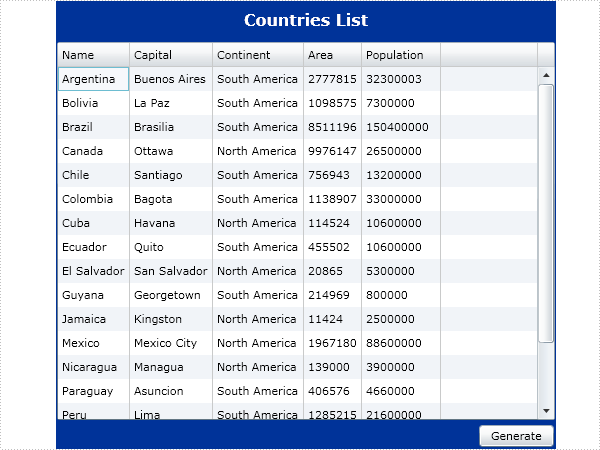Monday, 25 July 2011 09:12

### XLS to PDF in C#, VB.NET

The sample demonstrates how to convert Excel workbook to PDF file via Spire.XLS.

Get XLS-to-PDF.pdf.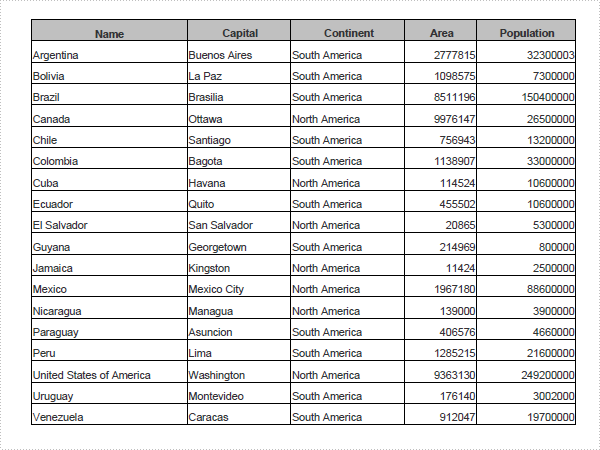Monday, 18 July 2011 06:43

### How to Export Datatable to Excel through DataGridView?

Export DataTable to Excel can be very easy through Spire.DataExport (or Spire.Office). Furthermore, Spire.DataExport enables user to Export data to Excel through DataGridView. Through DataGridView users can preview and modify data information before exporting.

Download Spire.DataExport (or Spire.Office) with .NET Framework together. Only 2 Simple steps you can finish the whole datatable to Excel exporting process.

In this step, Spire.DataExport will help you load Data information from your datatable. After you put in your data source and SQL command, you can preview and modify data information in DataGridView area.

[C#]
```
private void btnLoad_Click(object sender, EventArgs e)
{
using(OleDbConnection oleDbConnection = new OleDbConnection())
{
oleDbConnection.ConnectionString = this.textBox1.Text;
OleDbCommand oleDbCommand = new OleDbCommand();
oleDbCommand.CommandText = this.textBox2.Text;
oleDbCommand.Connection = oleDbConnection;
{
DataTable dt = new DataTable();
da.Fill(dt);
dataGridView1.DataSource = dt;
}
}
}

```

Effect Screenshot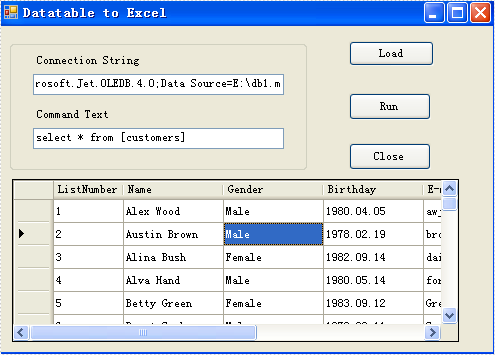Step 2: Set Export into Excel

Spire.DataExport allows user to export data into most popular file formats including MS Excel, MS Word, HTML, PDF, XML, CSV, DBF, DIF, etc. Now, in this step you should give an order to Export Data into Excel file format. Spire.DataExport will create a new MS Excel Worksheet for storing Data which exported out. You can rename the excel file in this step either.

[C#]
```        private void btnRun_Click(object sender, EventArgs e)
{
Spire.DataExport.XLS.CellExport cellExport = new Spire.DataExport.XLS.CellExport();
Spire.DataExport.XLS.WorkSheet worksheet1 = new Spire.DataExport.XLS.WorkSheet();
worksheet1.DataSource = Spire.DataExport.Common.ExportSource.DataTable;
worksheet1.DataTable = this.dataGridView1.DataSource as DataTable;
worksheet1.StartDataCol = ((System.Byte)(0));
cellExport.ActionAfterExport = Spire.DataExport.Common.ActionType.OpenView;
cellExport.SaveToFile("20110223.xls");
}

```

Effect Screenshot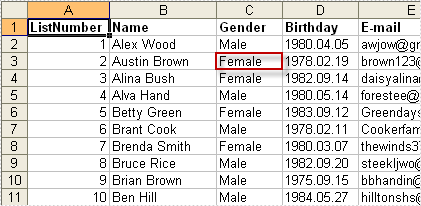Monday, 18 July 2011 06:39

### Spire.DataExport Program Guide Content

Spire.DataExport, a professional .NET DataExport library, enables users to export data from database, datatable and other datasoure to MS Excel, MS Word, PDF, MS Access, DBF, SQL Script, SYLK, DIF, CSV and MS Clipboard without MS Office, Adobe Acrobat and other third-party installed on system. With Spire.DataExport, developer can create any WinForm and Web applications to export data.

Spire.DataExport is fantastic on formatting when exporting, especially for Excel. Users can format data, import formula, insert objects (image, hyperlinks etc.) and create chart when export a large amount of data to multiple worksheets. The following sections will present how to quickly export data in details.

Tuesday, 12 July 2011 06:48

### Doc MailMerge Silverlight

The sample demonstrates how to work with MailMerge in Silverlight via Spire.Doc.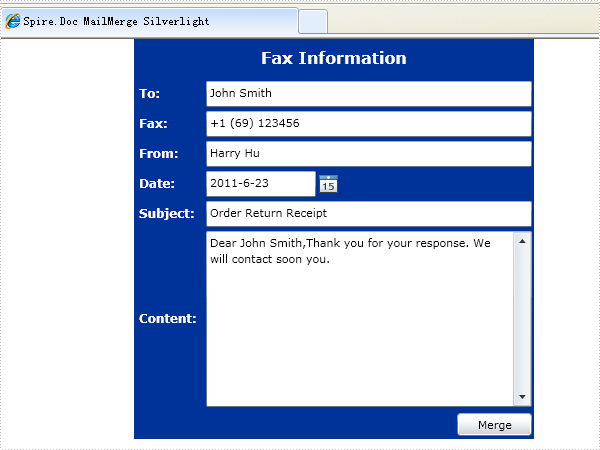Wednesday, 18 May 2011 10:12

### How to Use PDFConverter to Encrypt PDF Document

Spire.PDFConverter enables users to encrypt PDF documents when converting to PDF. Users can set own password and user password. Also, users can set individual security permissions, for example, print, edit and so on.

### How to Encrypt PDF Document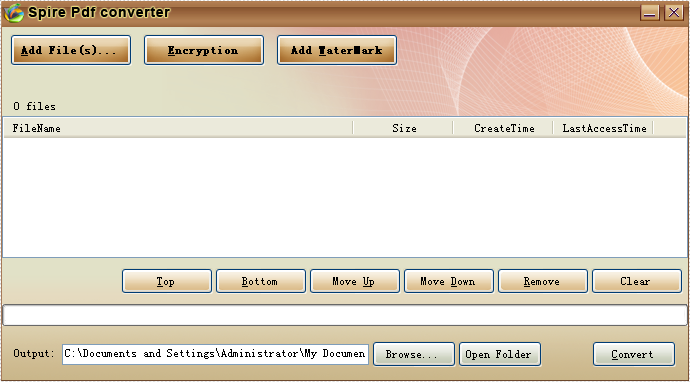Step 2: Choose a Document.

Click Add files, select the document you want to convert and encrypt.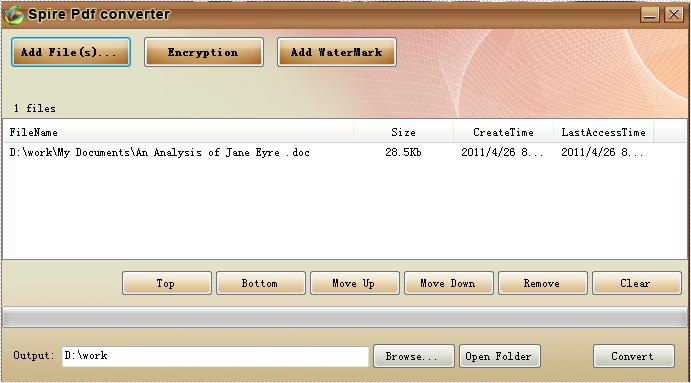Step 3: Encrypt PDF Document

Click Encryption button and then set password and permissions.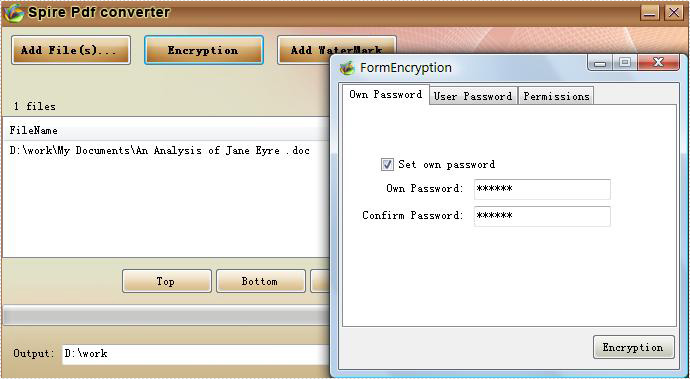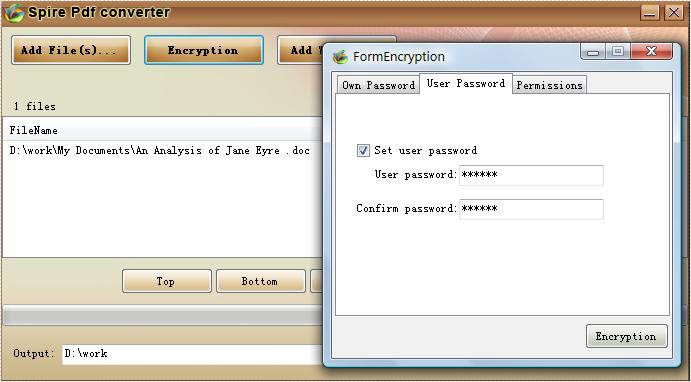Permissions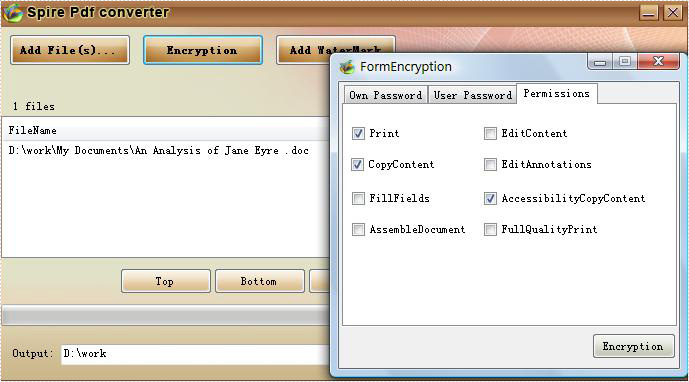Step 4: Convert Document

Click Encryption to confirm. Save the document in a specific path by clicking browse. Then, Click Convert.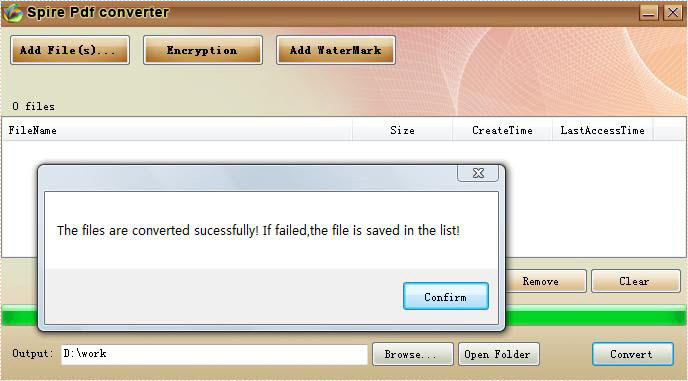Now, the document has been converted to PDF and encrypted.

Page 5 of 22